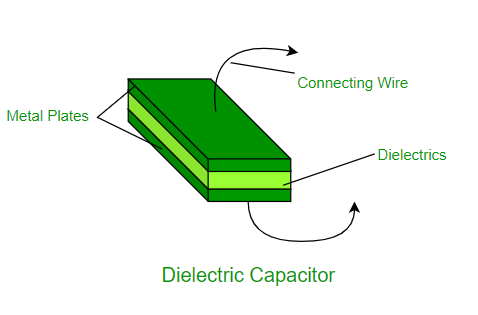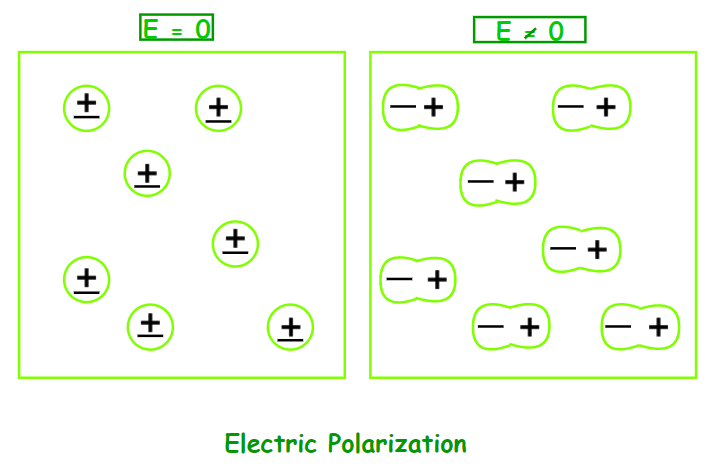Open in App
Not now

# Dielectrics and Polarisation

• Last Updated : 16 Nov, 2022

Have you noticed how many of the insulators are made of wood, plastic, or glass? But why is that? When we utilise wood or plastic, why don’t we receive electric shocks? Why do you only get severe shocks from metal wires? We’ll look at dielectrics, polarisation, the dielectric constant, and more in this chapter. We’ll look at some typical applications and examples of these dielectrics as well.

### What is a Dielectric?

Non-conducting materials are known as dielectrics. They are insulating materials and poor electric current conductors. Dielectric materials can maintain an electrostatic charge while losing very little energy as heat. Mica, plastics, glass, porcelain, and other metal oxides are examples of dielectrics. It’s also important to note that even dry air is dielectric.Classification of Dielectrics

Dielectrics are of two types:

• Polar Molecules: Polar Molecules are those types of dielectrics in which the chances of positive and negative molecules colliding are nil or zero. This is because they are all asymmetrical in form. H2O, CO2, NO2, and other gases are examples. When there is no electric field, the electric dipole moment of these molecules moves in an unpredictable direction. The average dipole moment is 0 as a result of this. If there is an external electric field, the molecules will assemble in the same direction as the electric field.
• Non-Polar Molecule: Unlike polar molecules, the centre of positive and negative charge in non-polar molecules does not coincide, i.e., zero. There is no longer any persistent (or inherent) dipole moment in the molecule. Examples include O2, N2, H2, and others.

### Induced Electric Dipole Moment

When an external electric field is applied to a non-polar molecule, all protons move in the same direction as the electric field, while electrons move in the opposite direction. This process will continue unless the internal pressures are balanced due to the existence of an electric field. This results in the formation of two charge centres. We name them the Induced Electric Dipole because they are polarised. The Induced Electric Dipole Moment is the dipole moment.

Polarizability

When the matter is exposed to an electric field, it has a propensity to develop an electric dipole moment that is proportional to the applied field. Because matter is made up of fundamental particles with an electric charge, such as protons and electrons, it is a property of all matter.

Negatively charged electrons and positively charged atomic nuclei are subjected to opposing forces and undergo charge separation when exposed to an electric field. The dielectric constant and, at high (optical) frequencies, the refractive index of a material is determined by its polarizability.

The applied field is proportional to the induced dipole moment and is temperature independent. For a single polar atom, the direction of induced dipole moment (x) is parallel to the direction of electric field E. The polarisability of a bound system influences its dynamical responsiveness to external fields.

It also gives information about a molecule’s interior structure. Polarizability is a solid is defined as the dipole moment per unit volume of the crystal cell:

P = ε0 α E

where α is the atomic polarizability & E is the electric field.

Its S.I. unit is m3 and its dimensions are the same as its volume.

Electric Polarization

When a dielectric slab is placed in an electric field, the molecule acquires a dipole moment. In such circumstances, the dielectric is said to be polarised. The Electric Polarization of a dielectric substance is the dipole moment per unit volume. P is the symbol for polarisation.### Dielectric Constant

The Dielectric Constant is the ratio of the applied electric field strength to the strength of the decreased value of the electric field capacitor when a dielectric slab is placed between the parallel plates. The formula is as follows:

εr = E0 / E

where E0 is the applied electric field, E is the net field, & εr is the dielectric constant.

The greater the dielectric constant, the greater the amount of charge that can be held. The capacitance of a capacitor is increased by a factor of the dielectric constant when the gap between the plates is completely filled with a dielectric. C = εr C0, where C0 is the capacitance between the plates with no dielectric.

Dielectric Strength

The dielectric strength of insulating material is the highest electric field strength that it can tolerate intrinsically without losing its insulating characteristics.

### Dielectric Polarization

Dielectric Polarization occurs when an external electric field is applied to a dielectric substance. When an electric field is applied, it causes charges (both positive and negative) to be displaced. The primary goal of dielectric polarisation is to connect macroscopic and microscopic characteristics.

In the case of piezoelectric crystals, polarisation is caused by the action of an electric field or other external variables such as mechanical stress. Solid materials that collect electric charge are known as piezoelectric crystals.

Dielectric In pyroelectric crystals, polarisation can also occur spontaneously, especially in ferroelectrics. Ferroelectricity is a feature of some materials in which the spontaneous electric polarisation may be reversed by applying an electric field.

The formula for polarization is given as:

P =  ε0 χe E = ε0r − 1) E

where P is the polarization, χe is the susceptibility, & E is the electric field.

### Sample Questions

Question 1: Electric field inside the capacitor is 50 V/m and the dielectric constant = 4.5. What is polarization?

Given:

Dielectric Constant, εr = 4.5

Electric Field, E = 50 V/m

Susceptibilty, χe = εr − 1 = 4.5 − 1 = 3.5

Polarization, P = χe E

= 3.5 × 50

= 175 C/m2

Hence, the polarization is 175 C/m2.

Question 2: What is dielectric polarization?

When an external electric field is applied to a material, dielectric polarisation is the term used to describe its behaviour. An externally applied electric field causes a dipole moment to develop in an insulating material, which is known as dielectric polarisation.

When a current interacts with a dielectric (insulating) substance, the dielectric material shifts its charge distribution, with positive charges aligning with the electric field and negative charges aligning with the electric field. Important circuit components like capacitors may be manufactured by taking use of this reaction.

Question 3: The relative dielectric constant of polystyrene is 3.5. What is the polarization produced when a 1.5 mm thick sheet of polystyrene is subjected to 240 V?

Given that,

Dielectric constant,

εr = 3.5

ε0 = 8.85 × 10−12 C / V m

Thickness, d = 1.5 mm = 1.5 × 10−3 m

Voltage, V = 240 V

Electric Field, E = V/d

= 240 / (1.5 × 10-3) V/m

= 1.6 × 105 V/m

Polarization, P = ε0r − 1) E

= 8.85 × 10−12 × (3.5 – 1) × 1.5 × 105 C/m2

= 3.32 × 10−6 C/m2

Hence, the polarization produced in polystyrene is 3.32 × 10−6 C/m2.

Question 4: Explain insulators and their properties?

Insulators are materials that make it difficult for electric charges to flow freely. The outer shell electrons are firmly linked to the nucleus in the atoms of such substances. These chemicals have a high impedance to the passage of electricity because they lack free charge carriers. Glass, diamond, porcelain, plastic, nylon, wood, mica, and other non-metals are all insulators.

Properties:

• Insulators have a poor conductivity and a high resistance.
• Their atoms contain electrons that are firmly bonded and do not migrate throughout the substance.
• A current cannot readily flow because the electrons are stagnant and not freely moving.

Question 5: What are dielectric materials?